Next: Wave Propagation in Uniform Up: Magnetostatics in Magnetic Media Previous: Magnetic Energy

# Exercises

1. Given that the bound charge density associated with a polarization field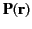is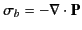, use charge conservation to deduce that the current density due to bound charges is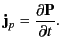2. Given that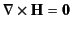in the absence of true currents, and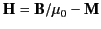, demonstrate that the current density due to magnetization currents is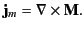3. A cylindrical hole of radius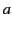is bored parallel to the axis of a cylindrical conductor of radius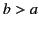which carries a uniformly distributed current of density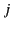running parallel to its axis. The distance between the center of the conductor and the center of the hole is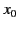. Find the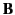field in the hole.

4. A sphere of radiuscarries a uniform surface charge density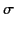. The sphere is rotated about a diameter with constant angular velocity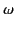. Find the vector potential and thefield both inside and outside the sphere.

5. Find theand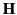fields inside and outside a spherical shell of inner radiusand outer radius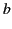which is magnetized permanently to a constant magnetization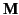.

6. A long hollow, right cylinder of inner radiusand outer radius, and of relative permeability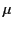, is placed in a region of initially uniform magnetic flux densityat right-angles to the field. Find the flux density at all points in space. Neglect end effects.

7. A transformer consists of a thin uniform ring of ferromagnetic material of radius, cross-sectional area, and magnetic permeability. The primary circuit is wrapped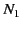times around one side of the ring, and the secondary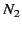times around the other side. Show that the mutual inductance between the two circuits is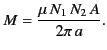Suppose that a thin gap of thickness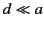is cut in a part of the ring in which there are no windings. What is the new mutual inductance of the two circuits? Suppose that the gap is filled with ferromagnetic material of permeability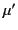. What, now, is the mutual inductance of the circuits? You may neglect flux-leakage (i.e., you may assume that magnetic field-lines do not leak out of the transformer core into the surrounding vacuum, except in the gap).Next: Wave Propagation in Uniform Up: Magnetostatics in Magnetic Media Previous: Magnetic Energy
Richard Fitzpatrick 2014-06-27